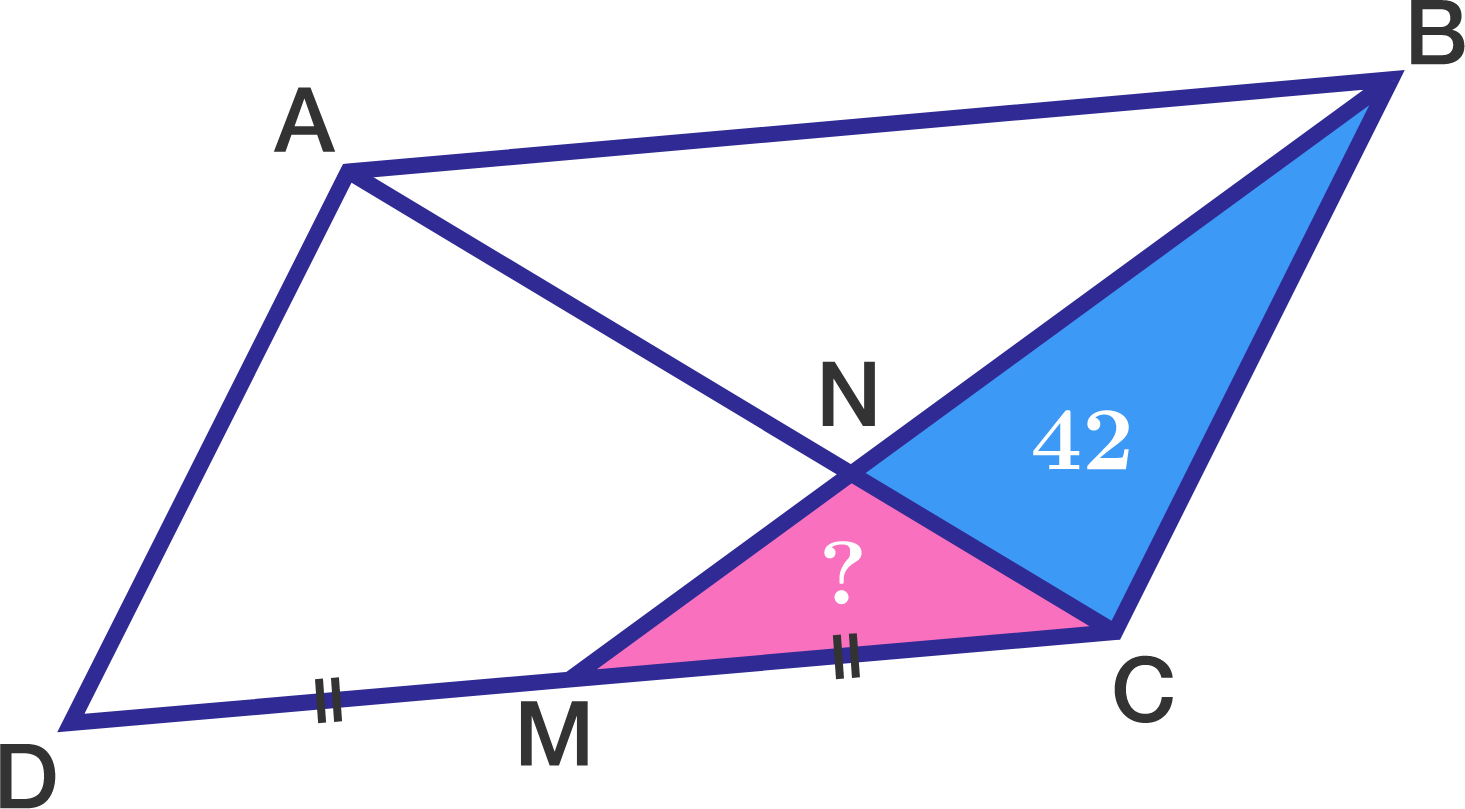# Geometry problem #60059

Geometry Level 1In the diagram to the right, quadrilateral $ABCD$ is a parallelogram and $M$ is the midpoint of $\overline{DC}.$

If the area of $\triangle BNC$ is $42,$ what is the area of $\triangle MNC?$

×